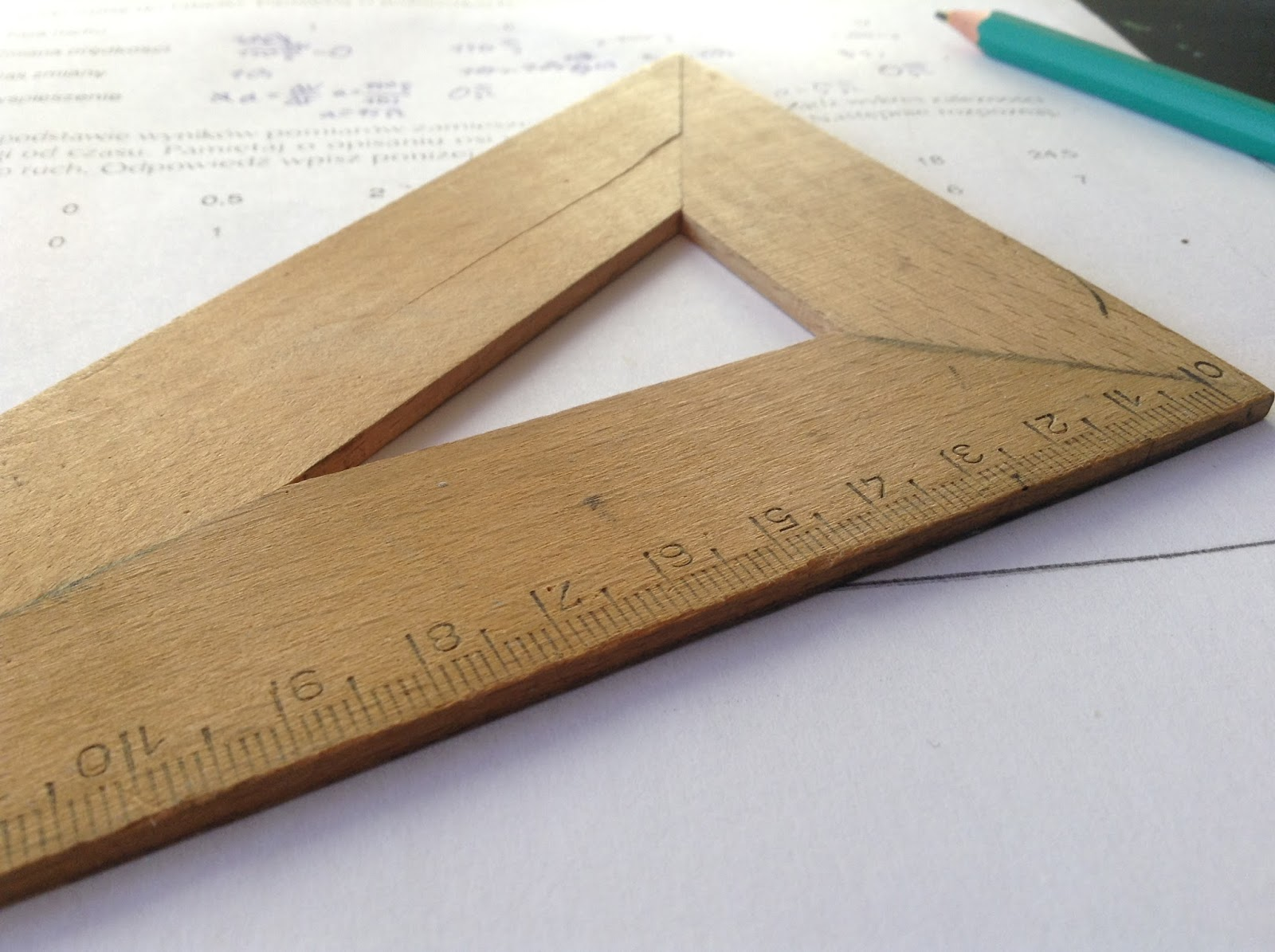Fri. Dec 1st, 2023

Jan 24, 2018

# Trigonometry Formulas### Trigonometry Formulas

If we noticed very carefully in any triangle, there is some relation between the length of the side and the angle of a triangle. Trigonometry is that branch of mathematics where we can plug the relation between them in a very systematic and simple way. Now a days it has many applications in field mathematics or in engineering fields (mostly Civil Engineering, Mechanical Engineering, Architectural Engineering).So, according to me, the studies of Trigonometry are very essential for students. If you learn this topic very sincerely and positively I can assure you that soon you’ll become strong in mathematics.

Subscribe my Youtube Channel : Science Duniya in Bangla

#### Parul Target Madhyamik 2024: English Model Set 1

Insert math as
$${}$$